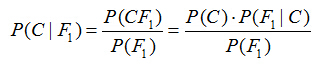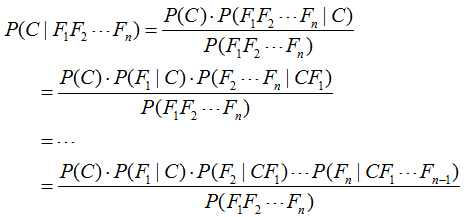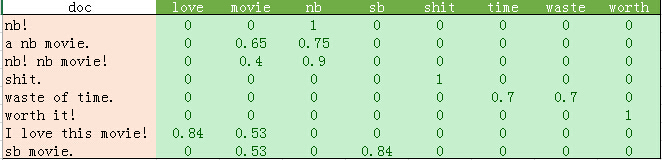# 用Python开始机器学习（6：朴素贝叶斯分类器）

## 1、贝叶斯定理## 2、朴素的概念

P(C)*P(F1|C)*P(F2|C)...P(Fn|C)。

log[P(C)*P(F1|C)*P(F2|C)...P(Fn|C)] = log[P(C)]+log[P(F1|C)] + ... +log[P(Fn|C)]P(C=0)=3/8， P(C=1)=5/8。特征F1="nb", F2="movie"。

（注意：实际计算中还要考虑上表中各个值的TF-IDF，具体计算方式取决于使用哪一类贝叶斯分类器。分类器种类见本文最后说明）

## 3、测试数据

#保存
sp.save('movie_data.npy', movie_data)
sp.save('movie_target.npy', movie_target)

#读取
movie_target = sp.load('movie_target.npy')

## 4、代码与分析

Python代码如下：

# -*- coding: utf-8 -*-
from matplotlib import pyplot
import scipy as sp
import numpy as np
from sklearn.datasets import load_files
from sklearn.cross_validation import train_test_split
from sklearn.feature_extraction.text import  CountVectorizer
from sklearn.feature_extraction.text import  TfidfVectorizer
from sklearn.naive_bayes import MultinomialNB
from sklearn.metrics import precision_recall_curve
from sklearn.metrics import classification_report

'''
#保存
sp.save('movie_data.npy', movie_reviews.data)
sp.save('movie_target.npy', movie_reviews.target)
'''

#读取
x = movie_data
y = movie_target

#BOOL型特征下的向量空间模型，注意，测试样本调用的是transform接口
count_vec = TfidfVectorizer(binary = False, decode_error = 'ignore',\
stop_words = 'english')

#加载数据集，切分数据集80%训练，20%测试
x_train, x_test, y_train, y_test\
= train_test_split(movie_data, movie_target, test_size = 0.2)
x_train = count_vec.fit_transform(x_train)
x_test  = count_vec.transform(x_test)

#调用MultinomialNB分类器
clf = MultinomialNB().fit(x_train, y_train)
doc_class_predicted = clf.predict(x_test)

#print(doc_class_predicted)
#print(y)
print(np.mean(doc_class_predicted == y_test))

#准确率与召回率
precision, recall, thresholds = precision_recall_curve(y_test, clf.predict(x_test))
report = answer > 0.5
print(classification_report(y_test, report, target_names = ['neg', 'pos']))

0.821428571429
precision    recall  f1-score   support
neg       0.78      0.87      0.83       135
pos       0.87      0.77      0.82       145
avg / total     0.83      0.82      0.82       280

03-153914
09-20
12-113547
12-301692
01-041604
04-153119
10-1694
12-102539
06-152686
11-01460
08-166万+
02-05713
03-05968
07-04593
03-27410
08-01363
10-081772
06-052197
02-051320
10-231546

### “相关推荐”对你有帮助么？

•非常没帮助
•没帮助
•一般
•有帮助
•非常有帮助

©️2022 CSDN 皮肤主题：大白 设计师：CSDN官方博客被折叠的  条评论 为什么被折叠?到【灌水乐园】发言lsldd

¥2 ¥4 ¥6 ¥10 ¥20余额支付 (余额：-- )扫码支付获取中扫码支付点击重新获取扫码支付1.余额是钱包充值的虚拟货币，按照1:1的比例进行支付金额的抵扣。
2.余额无法直接购买下载，可以购买VIP、C币套餐、付费专栏及课程。余额充值